# Train Deep Learning Semantic Segmentation Network Using 3-D Simulation Data

This example shows how to use 3-D simulation data to train a semantic segmentation network and fine-tune it to real-world data using generative adversarial networks (GANs).

This example uses 3-D simulation data generated by Driving Scenario Designer and the Unreal Engine®. For an example showing how to generate such simulation data, see Depth and Semantic Segmentation Visualization Using Unreal Engine Simulation (Automated Driving Toolbox). The 3-D simulation environment generates the images and the corresponding ground truth pixel labels. Using the simulation data avoids the annotation process, which is both tedious and requires a large amount of human effort. However, domain shift models trained on only simulation data do not perform well on real-world data sets. To address this, you can use domain adaptation to fine-tune the trained model to work on a real-world data set.

This example uses AdaptSegNet , a network that adapts the structure of the output segmentation predictions, which look alike irrespective of the input domain. The AdaptSegNet network is based on the GAN model and consists of two networks that are trained simultaneously to maximize the performance of both:

1. Generator — Network trained to generate high-quality segmentation results from real or simulated input images

2. Discriminator — Network that compares and attempts to distinguish whether the segmentation predictions of the generator are from real or simulated data

To fine-tune the AdaptSegNet model for real-world data, this example uses a subset of the CamVid data  and adapts the model to generate high-quality segmentation predictions on the CamVid data.

Download the pretrained network. The pretrained model allows you to run the entire example without having to wait for training to complete. If you want to train the network, set the `doTraining` variable to `true`.

```doTraining = false; if ~doTraining pretrainedURL = 'https://ssd.mathworks.com/supportfiles/vision/data/trainedAdaptSegGANNet.mat'; pretrainedFolder = fullfile(tempdir,'pretrainedNetwork'); pretrainedNetwork = fullfile(pretrainedFolder,'trainedAdaptSegGANNet.mat'); if ~exist(pretrainedNetwork,'file') mkdir(pretrainedFolder); disp('Downloading pretrained network (57 MB)...'); websave(pretrainedNetwork,pretrainedURL); end pretrained = load(pretrainedNetwork); dlnetGenerator = pretrained.dlnetGenerator; end ```

Download the simulation and real data sets by using the `downloadDataset` function, defined in the Supporting Functions section of this example. The `downloadDataset` function downloads the entire CamVid data set and partition the data into training and test sets.

The simulation data set was generated by Driving Scenario Designer. The generated scenarios, which consist of 553 photorealistic images with labels, were rendered by the Unreal Engine. You use this data set to train the model.

The real data set is a subset of the CamVid data set from the University of Cambridge. To adapt the model to real-world data, 69 CamVid images. To evaluate the trained model, you use 368 CamVid images.

```simulationDataURL = 'https://ssd.mathworks.com/supportfiles/vision/data/SimulationDrivingDataset.zip'; realImageDataURL = 'http://web4.cs.ucl.ac.uk/staff/g.brostow/MotionSegRecData/files/701_StillsRaw_full.zip'; realLabelDataURL = 'http://web4.cs.ucl.ac.uk/staff/g.brostow/MotionSegRecData/data/LabeledApproved_full.zip'; simulationDataLocation = fullfile(tempdir,'SimulationData'); realDataLocation = fullfile(tempdir,'RealData'); [simulationImagesFolder, simulationLabelsFolder, realImagesFolder, realLabelsFolder, ... realTestImagesFolder, realTestLabelsFolder] = ... downloadDataset(simulationDataLocation,simulationDataURL,realDataLocation,realImageDataURL,realLabelDataURL);```

The downloaded files include the pixel labels for the real domain, but note that you do not use these pixel labels in the training process. This example uses the real domain pixel labels only to calculate the mean intersection over union (IoU) value to evaluate the efficacy of the trained model.

### Load Simulation and Real Data

Use `imageDatastore` to load the simulation and real data sets for training. By using an image datastore, you can efficiently load a large collection of images on disk.

```simData = imageDatastore(simulationImagesFolder); realData = imageDatastore(realImagesFolder);```

Preview images from the simulation data set and real data set.

```simImage = preview(simData); realImage = preview(realData); montage({simImage,realImage})```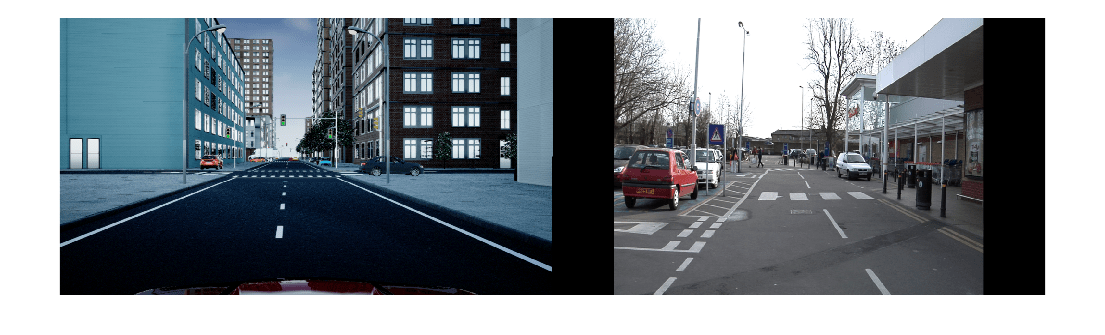The real and simulated images look very different. Consequently, models trained on simulated data and evaluated on real data perform poorly due to domain shift.

#### Load Pixel-Labeled Images for Simulation Data and Real Data

Load the simulation pixel label image data by using `pixelLabelDatastore` (Computer Vision Toolbox). A pixel label datastore encapsulates the pixel label data and the label ID to a class name mapping.

For this example, specify five classes useful for an automated driving application: road, background, pavement, sky, and car.

```classes = [ "Road" "Background" "Pavement" "Sky" "Car" ]; numClasses = numel(classes);```

The simulation data set has eight classes. Reduce the number of classes from eight to five by grouping the building, tree, traffic signal, and light classes from the original data set into a single background class. Return the grouped label IDs by using the helper function `simulationPixelLabelIDs`. This helper function is attached to the example as a supporting file.

`labelIDs = simulationPixelLabelIDs;`

Use the classes and label IDs to create a pixel label datastore of the simulation data.

`simLabels = pixelLabelDatastore(simulationLabelsFolder,classes,labelIDs);`

Initialize the colormap for the segmented images using the helper function `domainAdaptationColorMap`, defined in the Supporting Functions section.

`dmap = domainAdaptationColorMap;`

Preview a pixel-labeled image by overlaying the label on top of the image using the `labeloverlay` (Image Processing Toolbox) function.

```simImageLabel = preview(simLabels); overlayImageSimulation = labeloverlay(simImage,simImageLabel,'ColorMap',dmap); figure imshow(overlayImageSimulation) labelColorbar(dmap,classes);```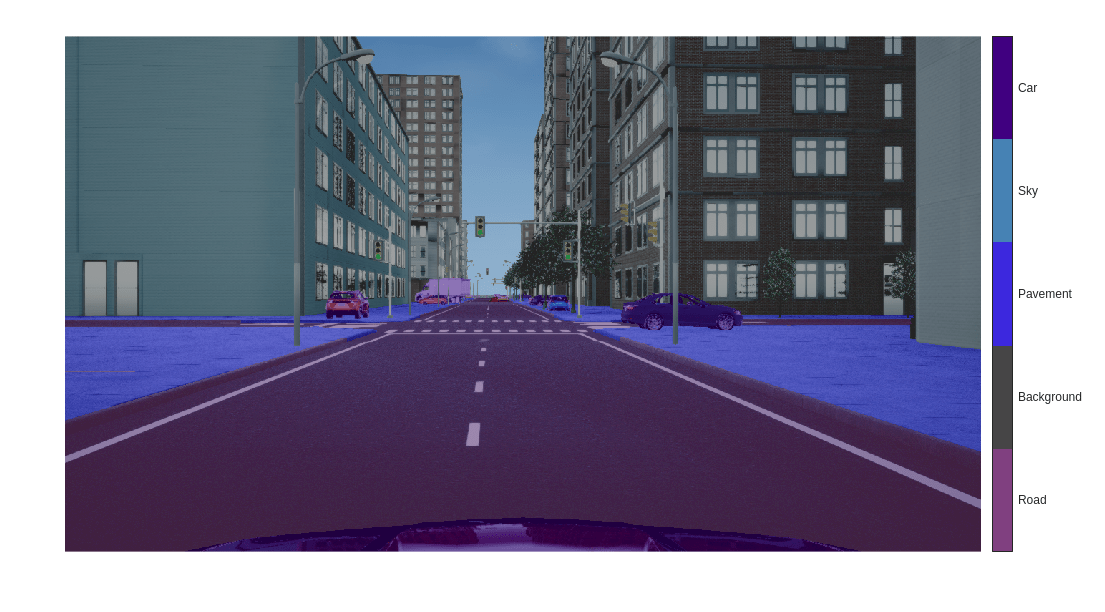Shift the simulation and real data used for training to zero center, to center the data around the origin, by using the `transform` function and the `preprocessData` helper function, defined in the Supporting Functions section.

```preprocessedSimData = transform(simData, @(simdata)preprocessData(simdata)); preprocessedRealData = transform(realData, @(realdata)preprocessData(realdata));```

Use the `combine` function to combine the transformed image datastore and pixel label datastores of the simulation domain. The training process does not use the pixel labels of real data.

`combinedSimData = combine(preprocessedSimData,simLabels);`

This example modifies the VGG-16 network pretrained on ImageNet to a fully convolutional network. To enlarge the receptive fields, dilated convolutional layers with strides of 2 and 4 are added. This makes the output feature map resolution one-eighth of the input size. Atrous spatial pyramid pooling (ASPP) is used to provide multiscale information and is followed by a `resize2dlayer` with an upsampling factor of 8 to resize the output to the size of the input.

The AdaptSegNet generator network used in this example is illustrated in the following diagram.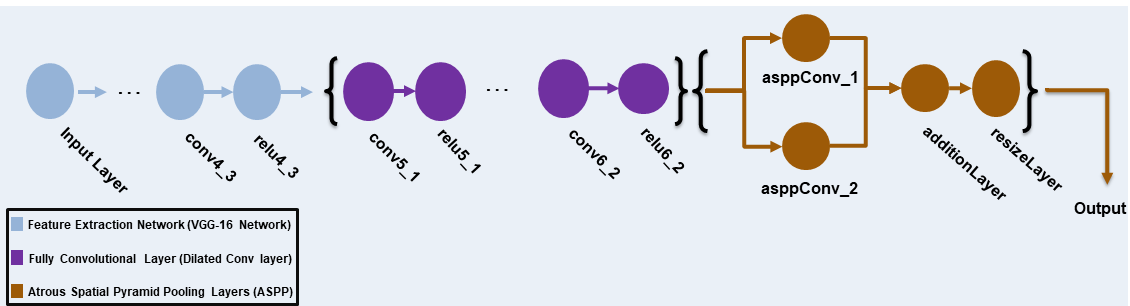To get a pretrained VGG-16 network, install the `vgg16`. If the support package is not installed, then the software provides a download link.

`net = vgg16;`

To make the VGG-16 network suitable for semantic segmentation, remove all VGG layers after `'relu4_3'`.

`vggLayers = net.Layers(2:24);`

Create an image input layer of size 1280-by-720-by-3 for the generator.

```inputSizeGenerator = [1280 720 3]; inputLayer = imageInputLayer(inputSizeGenerator,'Normalization','None','Name','inputLayer');```

Create fully convolutional network layers. Use dilation factors of 2 and 4 to enlarge the respective fields.

```fcnlayers = [ convolution2dLayer([3 3], 360,'DilationFactor',[2 2],'Padding',[2 2 2 2],'Name','conv5_1','WeightsInitializer','narrow-normal','BiasInitializer','zeros') reluLayer('Name','relu5_1') convolution2dLayer([3 3], 360,'DilationFactor',[2 2],'Padding',[2 2 2 2] ,'Name','conv5_2','WeightsInitializer','narrow-normal','BiasInitializer','zeros') reluLayer('Name','relu5_2') convolution2dLayer([3 3], 360,'DilationFactor',[2 2],'Padding',[2 2 2 2],'Name','conv5_3','WeightsInitializer','narrow-normal','BiasInitializer','zeros') reluLayer('Name','relu5_3') convolution2dLayer([3 3], 480,'DilationFactor',[4 4],'Padding',[4 4 4 4],'Name','conv6_1','WeightsInitializer','narrow-normal','BiasInitializer','zeros') reluLayer('Name','relu6_1') convolution2dLayer([3 3], 480,'DilationFactor',[4 4],'Padding',[4 4 4 4] ,'Name','conv6_2','WeightsInitializer','narrow-normal','BiasInitializer','zeros') reluLayer('Name','relu6_2') ];```

Combine the layers and create the layer graph.

```layers = [ inputLayer vggLayers fcnlayers ]; lgraph = layerGraph(layers);```

ASPP is used to provide multiscale information. Add the ASPP module to the layer graph with a filter size equal to the number of channels by using the `addASPPToNetwork` helper function, defined in the Supporting Functions section.

`lgraph = addASPPToNetwork(lgraph, numClasses);`

Apply `resize2dLayer` with an upsampling factor of 8 to make the output match the size of the input.

```upSampleLayer = resize2dLayer('Scale',8,'Method','bilinear','Name','resizeLayer'); lgraphGenerator = addLayers(lgraph,upSampleLayer); lgraphGenerator = connectLayers(lgraphGenerator,'additionLayer','resizeLayer');```

Visualize the generator network in a plot.

```plot(lgraphGenerator) title("Generator")```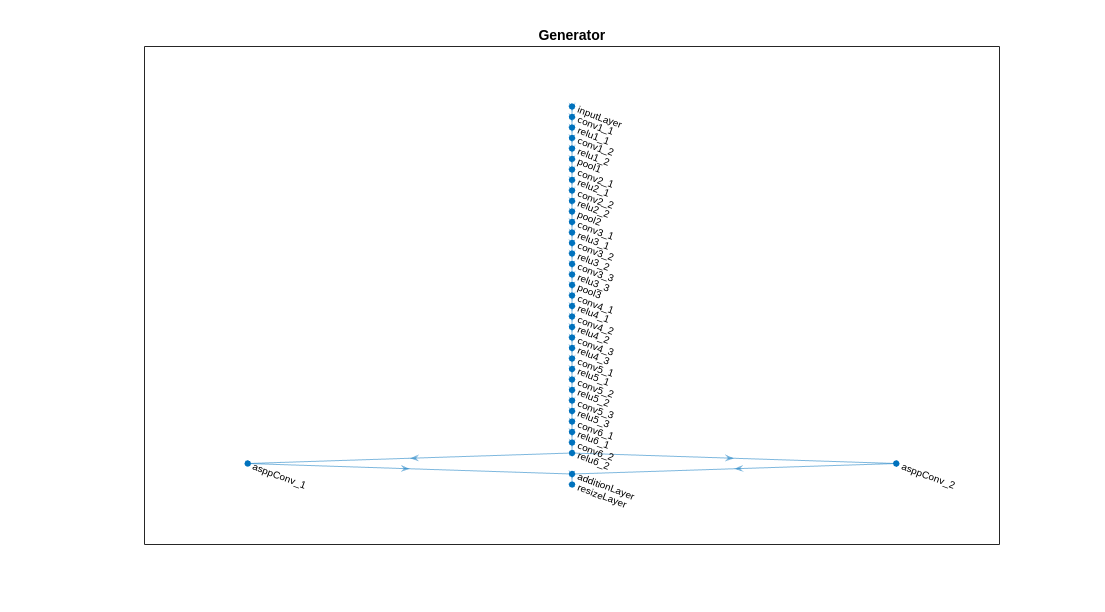The discriminator network consists of five convolutional layers with a kernel size of 3 and a stride of 2, where the number of channels is {64, 128, 256, 512, 1}. Each layer is followed by a leaky ReLU layer parameterized by a scale of 0.2, except for the last layer. `resize2dLayer` is used to resize the output of the discriminator. Note that this example does not use batch normalization, as the discriminator is jointly trained with the segmentation network using a small batch size.

The AdaptSegNet discriminator network in this example is illustrated in the following diagram.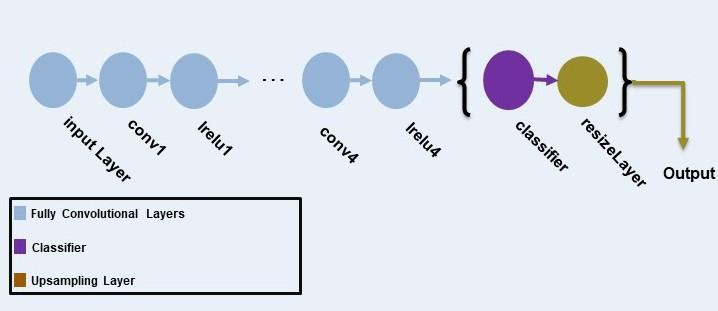Create an image input layer of size 1280-by-720-by-`numClasses` that takes in the segmentation predictions of the simulation and real domains.

`inputSizeDiscriminator = [1280 720 numClasses];`

Create fully convolutional layers and generate the discriminator layer graph.

```% Factor for number of channels in convolution layer. numChannelsFactor = 64; % Scale factor to resize the output of the discriminator. resizeScale = 64; % Scalar multiplier for leaky ReLU layers. leakyReLUScale = 0.2; % Create the layers of the discriminator. layers = [ imageInputLayer(inputSizeDiscriminator,'Normalization','none','Name','inputLayer') convolution2dLayer(3,numChannelsFactor,'Stride',2,'Padding',1,'Name','conv1','WeightsInitializer','narrow-normal','BiasInitializer','narrow-normal') leakyReluLayer(leakyReLUScale,'Name','lrelu1') convolution2dLayer(3,numChannelsFactor*2,'Stride',2,'Padding',1,'Name','conv2','WeightsInitializer','narrow-normal','BiasInitializer','narrow-normal') leakyReluLayer(leakyReLUScale,'Name','lrelu2') convolution2dLayer(3,numChannelsFactor*4,'Stride',2,'Padding',1,'Name','conv3','WeightsInitializer','narrow-normal','BiasInitializer','narrow-normal') leakyReluLayer(leakyReLUScale,'Name','lrelu3') convolution2dLayer(3,numChannelsFactor*8,'Stride',2,'Padding',1,'Name','conv4','WeightsInitializer','narrow-normal','BiasInitializer','narrow-normal') leakyReluLayer(leakyReLUScale,'Name','lrelu4') convolution2dLayer(3,1,'Stride',2,'Padding',1,'Name','classifer','WeightsInitializer','narrow-normal','BiasInitializer','narrow-normal') resize2dLayer('Scale', resizeScale,'Method','bilinear','Name','resizeLayer'); ]; % Create the layer graph of the discriminator. lgraphDiscriminator = layerGraph(layers);```

Visualize the discriminator network in a plot.

```plot(lgraphDiscriminator) title("Discriminator")```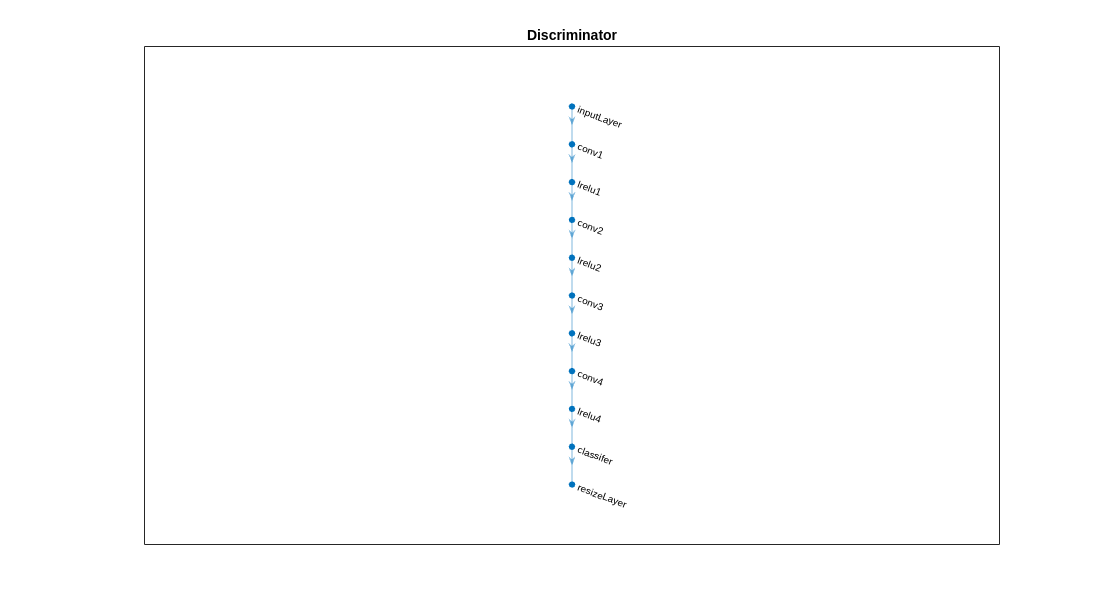### Specify Training Options

Specify these training options.

• Set the total number of iterations to `5000`. By doing so, you train the network for around 10 epochs.

• Set the learning rate for the generator to `2.5e-4`.

• Set the learning rate for the discriminator to `1e-4`.

• Set the L2 regularization factor to `0.0005`.

• The learning rate exponentially decreases based on the formula $learningrate×$ $\left[\frac{iteration}{total\phantom{\rule{0.2em}{0ex}}iterations}{\right]}^{power}$. This decrease helps to stabilize the gradients at higher iterations. Set the power to `0.9`.

• Set the weight of the adversarial loss to `0.001`.

• Initialize the velocity of the gradient as `[ ]`. This value is used by SGDM to store the velocity of the gradients.

• Initialize the moving average of the parameter gradients as `[ ]`. This value is used by Adam initializer to store the average of parameter gradients.

• Initialize the moving average of squared parameter gradients as `[ ]`. This value is used by Adam initializer to store the average of the squared parameter gradients.

• Set the mini-batch size to `1`.

```numIterations = 5000; learnRateGenBase = 2.5e-4; learnRateDisBase = 1e-4; l2Regularization = 0.0005; power = 0.9; lamdaAdv = 0.001; vel= []; averageGrad = []; averageSqGrad = []; miniBatchSize = 1;```

Train on a GPU, if one is available. Using a GPU requires Parallel Computing Toolbox™ and a CUDA® enabled NVIDIA® GPU. To automatically detect if you have a GPU available, set `executionEnvironment` to `"auto"`. If you do not have a GPU, or do not want to use one for training, set `executionEnvironment` to `"cpu"`. To ensure the use of a GPU for training, set `executionEnvironment` to `"gpu"`. For information about the supported compute capabilities, see GPU Computing Requirements (Parallel Computing Toolbox).

`executionEnvironment = "auto";`

Create the `minibatchqueue` object from the combined datastore of the simulation domain.

```mbqTrainingDataSimulation = minibatchqueue(combinedSimData,"MiniBatchSize",miniBatchSize, ... "MiniBatchFormat","SSCB","OutputEnvironment",executionEnvironment);```

Create the `minibatchqueue` object from the input image datastore of the real domain.

```mbqTrainingDataReal = minibatchqueue(preprocessedRealData,"MiniBatchSize",miniBatchSize, ... "MiniBatchFormat","SSCB","OutputEnvironment",executionEnvironment);```

### Train Model

Train the model using a custom training loop. The helper function `modelGradients`, defined in the Supporting Functions section of this example, calculate the gradients and losses for the generator and discriminator. Create the training progress plot using `configureTrainingLossPlotter`, attached to this example as a supporting file, and update the training progress using `updateTrainingPlots`. Loop over the training data and update the network parameters at each iteration.

For each iteration:

• Read the image and label information from the `minibatchqueue` object of the simulation data using the `next` function.

• Read the image information from the `minibatchqueue` object of the real data using the `next` function.

• Evaluate the model gradients using `dlfeval` and the `modelGradients` helper function, defined in the Supporting Functions section. `modelGradients` returns the gradients of the loss with respect to the learnable parameters.

• Update the generator network parameters using the `sgdmupdate` function.

• Update the discriminator network parameters using the `adamupdate` function.

• Update the training progress plot for every iteration and display various computed losses.

```if doTraining % Create the dlnetwork object of the generator. dlnetGenerator = dlnetwork(lgraphGenerator); % Create the dlnetwork object of the discriminator. dlnetDiscriminator = dlnetwork(lgraphDiscriminator); % Create the subplots for the generator and discriminator loss. fig = figure; [generatorLossPlotter, discriminatorLossPlotter] = configureTrainingLossPlotter(fig); % Loop through the data for the specified number of iterations. for iter = 1:numIterations % Reset the minibatchqueue of simulation data. if ~hasdata(mbqTrainingDataSimulation) reset(mbqTrainingDataSimulation); end % Retrieve the next mini-batch of simulation data and labels. [dlX,label] = next(mbqTrainingDataSimulation); % Reset the minibatchqueue of real data. if ~hasdata(mbqTrainingDataReal) reset(mbqTrainingDataReal); end % Retrieve the next mini-batch of real data. dlZ = next(mbqTrainingDataReal); % Evaluate the model gradients and loss using dlfeval and the modelGradients function. [gradientGenerator,gradientDiscriminator, lossSegValue, lossAdvValue, lossDisValue] = ... dlfeval(@modelGradients,dlnetGenerator,dlnetDiscriminator,dlX,dlZ,label,lamdaAdv); % Apply L2 regularization. gradientGenerator = dlupdate(@(g,w) g + l2Regularization*w, gradientGenerator, dlnetGenerator.Learnables); % Adjust the learning rate. learnRateGen = piecewiseLearningRate(iter,learnRateGenBase,numIterations,power); learnRateDis = piecewiseLearningRate(iter,learnRateDisBase,numIterations,power); % Update the generator network learnable parameters using the SGDM optimizer. [dlnetGenerator.Learnables, vel] = ... sgdmupdate(dlnetGenerator.Learnables,gradientGenerator,vel,learnRateGen); % Update the discriminator network learnable parameters using the Adam optimizer. [dlnetDiscriminator.Learnables, averageGrad, averageSqGrad] = ... adamupdate(dlnetDiscriminator.Learnables,gradientDiscriminator,averageGrad,averageSqGrad,iter,learnRateDis) ; % Update the training plot with loss values. updateTrainingPlots(generatorLossPlotter,discriminatorLossPlotter,iter, ... double(gather(extractdata(lossSegValue + lamdaAdv * lossAdvValue))),double(gather(extractdata(lossDisValue)))); end % Save the trained model. save('trainedAdaptSegGANNet.mat','dlnetGenerator'); end ```

The discriminator can now identify whether the input is from the simulation or real domain. In turn, the generator can now generate segmentation predictions that are similar across the simulation and real domains.

### Evaluate Model on Real Test Data

Evaluate the performance of the trained AdaptSegNet network by computing the mean IoU for the test data predictions.

Load the test data using `imageDatastore`.

`realTestData = imageDatastore(realTestImagesFolder);`

The CamVid data set has 32 classes. Use the `realpixelLabelIDs` helper function to reduce the number of classes to five, as for the simulation data set. The `realpixelLabelIDs` helper function is attached to this example as a supporting file.

`labelIDs = realPixelLabelIDs;`

Use `pixelLabelDatastore` (Computer Vision Toolbox) to load the ground truth label images for the test data.

`realTestLabels = pixelLabelDatastore(realTestLabelsFolder,classes,labelIDs);`

Shift the data to zero center to center the data around the origin, as for the training data, by using the `transform` function and the `preprocessData` helper function, defined in the Supporting Functions section.

`preprocessedRealTestData = transform(realTestData, @(realtestdata)preprocessData(realtestdata));`

Use `combine` to combine the transformed image datastore and pixel label datastores of the real test data.

`combinedRealTestData = combine(preprocessedRealTestData,realTestLabels);`

Create the `minibatchqueue` object from the combined datastore of the test data`. `Set `"MiniBatchSize"` to `1` for ease of evaluating the metrics.

```mbqimdsTest = minibatchqueue(combinedRealTestData,"MiniBatchSize",1,... "MiniBatchFormat","SSCB","OutputEnvironment",executionEnvironment);```

To generate the confusion matrix cell array, use the helper function `predictSegmentationLabelsOnTestSet` on `minibatchqueue` object of test data. The helper function `predictSegmentationLabelsOnTestSet` is listed below in Supporting Functions section.

`imageSetConfusionMat = predictSegmentationLabelsOnTestSet(dlnetGenerator,mbqimdsTest);`

Use `evaluateSemanticSegmentation` (Computer Vision Toolbox) to measure semantic segmentation metrics on the test set confusion matrix.

`metrics = evaluateSemanticSegmentation(imageSetConfusionMat,classes,'Verbose',false);`

To see the data set level metrics, inspect `metrics.DataSetMetrics`.

`metrics.DataSetMetrics`
```ans=1×4 table GlobalAccuracy MeanAccuracy MeanIoU WeightedIoU ______________ ____________ _______ ___________ 0.86883 0.769 0.64487 0.78026 ```

The data set metrics provide a high-level overview of network performance. To see the impact each class has on the overall performance, inspect the per-class metrics using `metrics.ClassMetrics`.

`metrics.ClassMetrics`
```ans=5×2 table Accuracy IoU ________ _______ Road 0.9147 0.81301 Background 0.93418 0.85518 Pavement 0.33373 0.27105 Sky 0.82652 0.81109 Car 0.83586 0.47399 ```

The data set performance is good, but the class metrics show that the car and pavement classes are not segmented well. Training the network using additional data can yield improved results.

### Segment Image

Run the trained network on one test image to check the segmented output prediction.

```% Read the image from the test data. data = readimage(realTestData,350); % Perform the preprocessing step of zero shift on the image. processeddata = preprocessData(data); % Convert the data to dlarray. processeddata = dlarray(processeddata,'SSCB'); % Predict the output of the network. [genPrediction, ~] = forward(dlnetGenerator,processeddata); % Get the label, which is the index with the maximum value in the channel dimension. [~, labels] = max(genPrediction,[],3); % Overlay the predicted labels on the image. segmentedImage = labeloverlay(data,uint8(gather(extractdata(labels))),'Colormap',dmap);```

Display the results.

```figure imshow(segmentedImage); labelColorbar(dmap,classes);```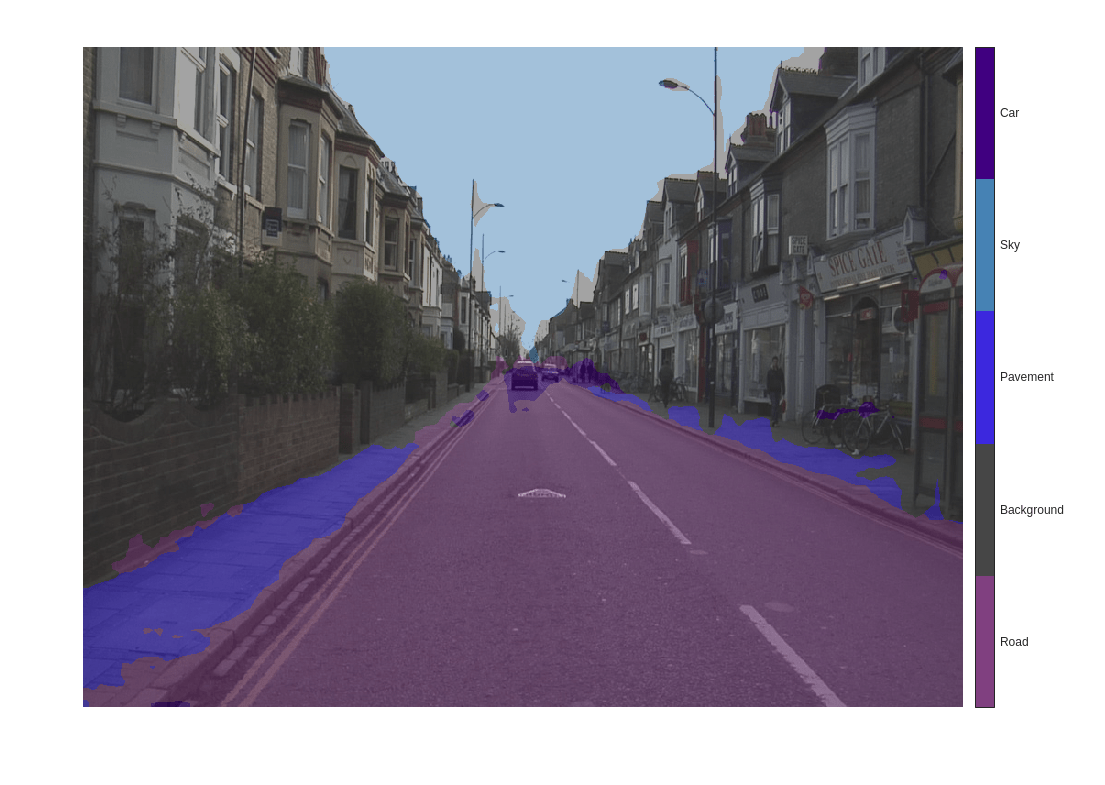Compare the label results with the expected ground truth stored in `realTestLabels`. The green and magenta regions highlight areas where the segmentation results differ from the expected ground truth.

```expectedResult = readimage(realTestLabels,350); actual = uint8(gather(extractdata(labels))); expected = uint8(expectedResult); figure imshowpair(actual,expected)```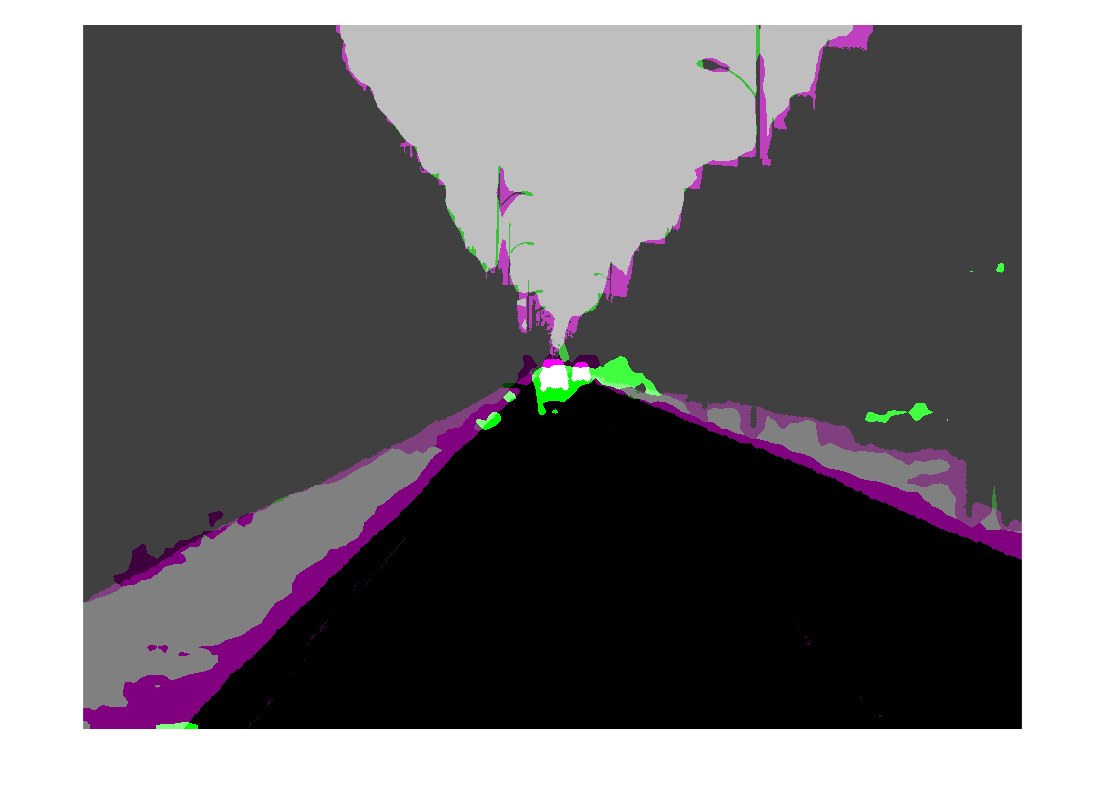Visually, the semantic segmentation results overlap well for the road, sky, and building classes. However, the results do not overlap well for the car and pavement classes.

### Supporting Functions

The helper function `modelGradients` calculates the gradients and adversarial loss for the generator and discriminator. The function also calculates the segmentation loss for the generator and the cross-entropy loss for the discriminator. As no state information is required to be remembered between the iterations for both generator and discriminator networks, the states are not updated.

```function [gradientGenerator, gradientDiscriminator, lossSegValue, lossAdvValue, lossDisValue] = modelGradients(dlnetGenerator, dlnetDiscriminator, dlX, dlZ, label, lamdaAdv) % Labels for adversarial training. simulationLabel = 0; realLabel = 1; % Extract the predictions of the simulation from the generator. [genPredictionSimulation, ~] = forward(dlnetGenerator,dlX); % Compute the generator loss. lossSegValue = segmentationLoss(genPredictionSimulation,label); % Extract the predictions of the real data from the generator. [genPredictionReal, ~] = forward(dlnetGenerator,dlZ); % Extract the softmax predictions of the real data from the discriminator. disPredictionReal = forward(dlnetDiscriminator,softmax(genPredictionReal)); % Create a matrix of simulation labels of real prediction size. Y = simulationLabel * ones(size(disPredictionReal)); % Compute the adversarial loss to make the real distribution close to the simulation label. lossAdvValue = mse(disPredictionReal,Y)/numel(Y(:)); % Compute the gradients of the generator with regard to loss. gradientGenerator = dlgradient(lossSegValue + lamdaAdv*lossAdvValue,dlnetGenerator.Learnables); % Extract the softmax predictions of the simulation from the discriminator. disPredictionSimulation = forward(dlnetDiscriminator,softmax(genPredictionSimulation)); % Create a matrix of simulation labels of simulation prediction size. Y = simulationLabel * ones(size(disPredictionSimulation)); % Compute the discriminator loss with regard to simulation class. lossDisValueSimulation = mse(disPredictionSimulation,Y)/numel(Y(:)); % Extract the softmax predictions of the real data from the discriminator. disPredictionReal = forward(dlnetDiscriminator,softmax(genPredictionReal)); % Create a matrix of real labels of real prediction size. Y = realLabel * ones(size(disPredictionReal)); % Compute the discriminator loss with regard to real class. lossDisValueReal = mse(disPredictionReal,Y)/numel(Y(:)); % Compute the total discriminator loss. lossDisValue = lossDisValueSimulation + lossDisValueReal; % Compute the gradients of the discriminator with regard to loss. gradientDiscriminator = dlgradient(lossDisValue,dlnetDiscriminator.Learnables); end```

### Segmentation Loss Function

The helper function `segmentationLoss` computes the feature segmentation loss, which is defined as the cross-entropy loss for the generator using the simulation data and its respective ground truth. The helper function computes the loss by using the `crossentropy` function.

```function loss = segmentationLoss(predict, target) % Generate the one-hot encodings of the ground truth. oneHotTarget = onehotencode(categorical(extractdata(target)),4); % Convert the one-hot encoded data to dlarray. oneHotTarget = dlarray(oneHotTarget,'SSBC'); % Compute the softmax output of the predictions. predictSoftmax = softmax(predict); % Compute the cross-entropy loss. loss = crossentropy(predictSoftmax,oneHotTarget,'TargetCategories','exclusive')/(numel(oneHotTarget)/2); end```

The helper function `downloadDataset` downloads both the simulation and real data sets from the specified URLs to the specified folder locations if they do not exist. The function returns the paths of the simulation, real training data, and real testing data. The function downloads the entire CamVid data set and partition the data into training and test sets using the `subsetCamVidDatasetFileNames` mat file, attached to the example as a supporting file.

```function [simulationImagesFolder, simulationLabelsFolder, realImagesFolder, realLabelsFolder,... realTestImagesFolder, realTestLabelsFolder] = ... downloadDataset(simulationDataLocation, simulationDataURL, realDataLocation, realImageDataURL, realLabelDataURL) % Build the training image and label folder location for simulation data. simulationDataZip = fullfile(simulationDataLocation,'SimulationDrivingDataset.zip'); % Get the simulation data if it does not exist. if ~exist(simulationDataZip,'file') mkdir(simulationDataLocation) disp('Downloading the simulation data'); websave(simulationDataZip,simulationDataURL); unzip(simulationDataZip,simulationDataLocation); end simulationImagesFolder = fullfile(simulationDataLocation,'SimulationDrivingDataset','images'); simulationLabelsFolder = fullfile(simulationDataLocation,'SimulationDrivingDataset','labels'); camVidLabelsZip = fullfile(realDataLocation,'CamVidLabels.zip'); camVidImagesZip = fullfile(realDataLocation,'CamVidImages.zip'); if ~exist(camVidLabelsZip,'file') || ~exist(camVidImagesZip,'file') mkdir(realDataLocation) disp('Downloading 16 MB CamVid dataset labels...'); websave(camVidLabelsZip, realLabelDataURL); unzip(camVidLabelsZip, fullfile(realDataLocation,'CamVidLabels')); disp('Downloading 587 MB CamVid dataset images...'); websave(camVidImagesZip, realImageDataURL); unzip(camVidImagesZip, fullfile(realDataLocation,'CamVidImages')); end % Build the training image and label folder location for real data. realImagesFolder = fullfile(realDataLocation,'train','images'); realLabelsFolder = fullfile(realDataLocation,'train','labels'); % Build the testing image and label folder location for real data. realTestImagesFolder = fullfile(realDataLocation,'test','images'); realTestLabelsFolder = fullfile(realDataLocation,'test','labels'); % Partition the data into training and test sets if they do not exist. if ~exist(realImagesFolder,'file') || ~exist(realLabelsFolder,'file') || ... ~exist(realTestImagesFolder,'file') || ~exist(realTestLabelsFolder,'file') mkdir(realImagesFolder); mkdir(realLabelsFolder); mkdir(realTestImagesFolder); mkdir(realTestLabelsFolder); % Load the mat file that has the names for testing and training. partitionNames = load('subsetCamVidDatasetFileNames.mat'); % Extract the test images names. imageTestNames = partitionNames.imageTestNames; % Remove the empty cells. imageTestNames = imageTestNames(~cellfun('isempty',imageTestNames)); % Extract the test labels names. labelTestNames = partitionNames.labelTestNames; % Remove the empty cells. labelTestNames = labelTestNames(~cellfun('isempty',labelTestNames)); % Copy the test images to the respective folder. for i = 1:size(imageTestNames,1) labelSource = fullfile(realDataLocation,'CamVidLabels',labelTestNames(i)); imageSource = fullfile(realDataLocation,'CamVidImages','701_StillsRaw_full',imageTestNames(i)); copyfile(imageSource{1}, realTestImagesFolder); copyfile(labelSource{1}, realTestLabelsFolder); end % Extract the train images names. imageTrainNames = partitionNames.imageTrainNames; % Remove the empty cells. imageTrainNames = imageTrainNames(~cellfun('isempty',imageTrainNames)); % Extract the train labels names. labelTrainNames = partitionNames.labelTrainNames; % Remove the empty cells. labelTrainNames = labelTrainNames(~cellfun('isempty',labelTrainNames)); % Copy the train images to the respective folder. for i = 1:size(imageTrainNames,1) labelSource = fullfile(realDataLocation,'CamVidLabels',labelTrainNames(i)); imageSource = fullfile(realDataLocation,'CamVidImages','701_StillsRaw_full',imageTrainNames(i)); copyfile(imageSource{1},realImagesFolder); copyfile(labelSource{1},realLabelsFolder); end end end```

The helper function `addASPPToNetwork` creates the atrous spatial pyramid pooling (ASPP) layers and adds them to the input layer graph. The function returns the layer graph with ASPP layers connected to it.

```function lgraph = addASPPToNetwork(lgraph, numClasses) % Define the ASPP dilation factors. asppDilationFactors = [6,12]; % Define the ASPP filter sizes. asppFilterSizes = [3,3]; % Extract the last layer of the layer graph. lastLayerName = lgraph.Layers(end).Name; % Define the addition layer. addLayer = additionLayer(numel(asppDilationFactors),'Name','additionLayer'); % Add the addition layer to the layer graph. lgraph = addLayers(lgraph,addLayer); % Create the ASPP layers connected to the addition layer % and connect the layer graph. for i = 1: numel(asppDilationFactors) asppConvName = "asppConv_" + string(i); branchFilterSize = asppFilterSizes(i); branchDilationFactor = asppDilationFactors(i); asspLayer = convolution2dLayer(branchFilterSize, numClasses,'DilationFactor', branchDilationFactor,... 'Padding','same','Name',asppConvName,'WeightsInitializer','narrow-normal','BiasInitializer','zeros'); lgraph = addLayers(lgraph,asspLayer); lgraph = connectLayers(lgraph,lastLayerName,asppConvName); lgraph = connectLayers(lgraph,asppConvName,strcat(addLayer.Name,'/',addLayer.InputNames{i})); end end```

The helper function `predictSegmentationLabelsOnTestSet` calculates the confusion matrix of the predicted and ground truth labels using the `segmentationConfusionMatrix` (Computer Vision Toolbox) function.

```function confusionMatrix = predictSegmentationLabelsOnTestSet(net, minbatchTestData) confusionMatrix = {}; i = 1; while hasdata(minbatchTestData) % Use next to retrieve a mini-batch from the datastore. [dlX, gtlabels] = next(minbatchTestData); % Predict the output of the network. [genPrediction, ~] = forward(net,dlX); % Get the label, which is the index with maximum value in the channel dimension. [~, labels] = max(genPrediction,[],3); % Get the confusion matrix of each image. confusionMatrix{i} = segmentationConfusionMatrix(double(gather(extractdata(labels))),double(gather(extractdata(gtlabels)))); i = i+1; end confusionMatrix = confusionMatrix'; end```

The helper function `piecewiseLearningRate` computes the current learning rate based on the iteration number.

```function lr = piecewiseLearningRate(i, baseLR, numIterations, power) fraction = i/numIterations; factor = (1 - fraction)^power * 1e1; lr = baseLR * factor; end```

The helper function `preprocessData` performs a zero center shift by subtracting the number of the image channels by the respective mean.

```function data = preprocessData(data) % Extract respective channels. rc = data(:,:,1); gc = data(:,:,2); bc = data(:,:,3); % Compute the respective channel means. r = mean(rc(:)); g = mean(gc(:)); b = mean(bc(:)); % Shift the data by the mean of respective channel. data = single(data) - single(shiftdim([r g b],-1)); end```

### References

 Tsai, Yi-Hsuan, Wei-Chih Hung, Samuel Schulter, Kihyuk Sohn, Ming-Hsuan Yang, and Manmohan Chandraker. “Learning to Adapt Structured Output Space for Semantic Segmentation.” In 2018 IEEE/CVF Conference on Computer Vision and Pattern Recognition, 7472–81. Salt Lake City, UT: IEEE, 2018. https://doi.org/10.1109/CVPR.2018.00780.

 Brostow, Gabriel J., Julien Fauqueur, and Roberto Cipolla. “Semantic Object Classes in Video: A High-Definition Ground Truth Database.” Pattern Recognition Letters 30, no. 2 (January 2009): 88–97. https://doi.org/10.1016/j.patrec.2008.04.005.